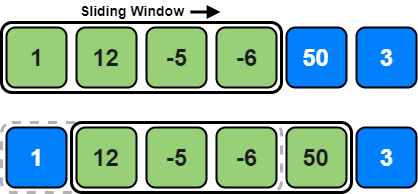# Coding Patterns: Sliding Window

In Coding Patterns series, we will try to recognize common patterns underlying behind each algorithm question, using real examples from Leetcode.

First, we will introduce Sliding Window pattern which is very useful to solve problems in which you are asked to find the longest/shortest string, subarray, or a desired value which you need to calculate from subarrays.

## Problem: Maximum Average Subarray

LeetCode 643 - Maximum Average Subarray I [easy]

Given an array consisting of `n` integers, find the contiguous subarray of given length `k` that has the maximum average value. And you need to output the maximum average value.

Example:

``````Input: [1, 12, -5, -6, 50, 3], k = 4
Output: 12.75
Explanation: Maximum average is (12 - 5 - 6 + 50) / 4 = 51 / 4 = 12.75
``````

### Brute Force Solution

``````class Solution:
def findMaxAverage(self, nums: List[int], k: int) -> float:
max_average = 0.0

for i in range(len(nums) - k + 1):
_sum = 0.0  # find sum of next k elements

for j in range(i, i + k):
_sum += nums[j]

average = _sum / k  # calculate the average of selected k elements
max_average = max(max_average, average)  # update max_average

if len(nums) == 1:  # if there is only 1 element in nums
return average

return max_average
``````

Time Complexity: O(N*k) where `N` is the number of elements in the input array.

This solution exceeds the time limit defined by Leetcode and is not accepted.

If we check our example array again: `[1, 12, -5, -6, 50, 3]` for `k = 4`, there are 3 overlapping elements between subarrays `[1, 12, -5, -6]` and `[12, -5, -6, 50]`. Can we somehow reuse the `_sum` we have calculated for the overlapping elements to make our solution more efficient?

### Sliding Window SolutionTo be able to reuse the `_sum` from the previous subarray, we will subtract the element going out of the window and add the element now being included in the sliding window.

``````class Solution:
def findMaxAverage(self, nums: List[int], k: int) -> float:
average = []
_sum, start = 0, 0
for end in range(len(nums)):
_sum += nums[end]  # add the next element

if end >= k - 1:
average.append(_sum / k)  # calculate the average
_sum -= nums[start]  # subtract the element going out
start += 1  # slide the window

return max(average)
``````

Time Complexity: O(N) where `N` is the number of elements in the input array.

## How to identify?

So we want to be able to identify the problems that sliding window pattern works.

• The problem involves a data structure that is ordered and iterable like arrays, strings, etc.
• The problem is asking to find a subrange in an array/string, contiguous longest, shortest, average or target value.
• There is an apparent naive or brute force solution that runs in O(N2), O(2N) or some other large time complexity.

The amazing thing about sliding window problems is that most of the time they can be solved in O(N) time and O(1) space complexity.

Tags:

Categories:

Updated: